DraftKings Super Bowl Odds
+550
5.5 to 1Buccaneers
12.7% implied probability

+650
6.5 to 1Chiefs
11.0% implied probability

+700
7 to 1Cardinals
10.3% implied probability

+700
7 to 1Patriots
10.3% implied probability

+750
7.5 to 1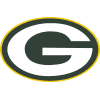Packers
9.7% implied probability

+1100
11 to 1Bills
6.9% implied probability

+1200
12 to 1Rams
6.4% implied probability

+1300
13 to 1Cowboys
5.9% implied probability

+1600
16 to 1Ravens
4.9% implied probability

+2200
22 to 1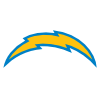Chargers
3.6% implied probability

+2500
25 to 1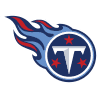Titans
3.2% implied probability

+3500
35 to 1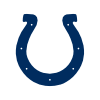Colts
2.3% implied probability

+3500
35 to 1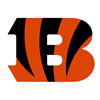Bengals
2.3% implied probability

+4000
40 to 1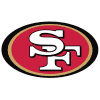49ers
2.0% implied probability

+6000
60 to 1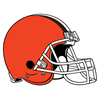Browns
1.4% implied probability

+8000
80 to 1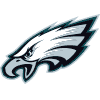Eagles
1.0% implied probability

+10000
100 to 1Football Team
0.8% implied probability

+10000
100 to 1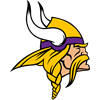Vikings
0.8% implied probability

+10000
100 to 1Broncos
0.8% implied probability

+10000
100 to 1Steelers
0.8% implied probability

+15000
150 to 1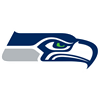Seahawks
0.5% implied probability

+15000
150 to 1Saints
0.5% implied probability

+20000
200 to 1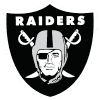Raiders
0.4% implied probability

+20000
200 to 1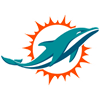Dolphins
0.4% implied probability

+50000
500 to 1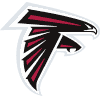Falcons
0.2% implied probability

+50000
500 to 1Panthers
0.2% implied probability

+100000
1000 to 1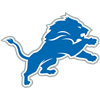Lions
0.1% implied probability

+100000
1000 to 1Jets
0.1% implied probability

+100000
1000 to 1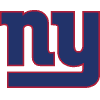Giants
0.1% implied probability

+100000
1000 to 1Jaguars
0.1% implied probability

+100000
1000 to 1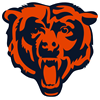Bears
0.1% implied probability